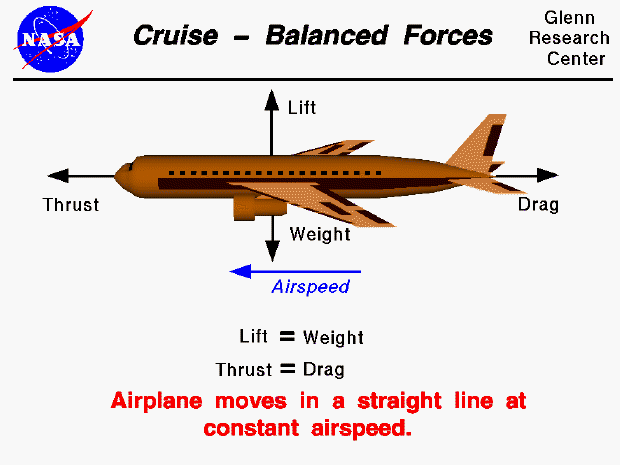There are four forces that act on an aircraft in flight: lift, weight, thrust, and drag. From Newton's first law of motion we know that an object at rest will stay at rest, and an object in motion (constant velocity) will stay in motion unless acted on by an external force. If there is no net external force, the object will maintain a constant velocity. In an ideal situation, the forces acting on an aircraft in flight can produce no net external force. In this situation the lift is equal to the weight, and the thrust is equal to the drag. The closest example of this condition is a cruising airliner. While the weight decreases due to fuel burned, the change is very small relative to the total aircraft weight. The aircraft maintains a constant airspeed called the cruise velocity.

If we take into account the relative velocity of the wind, we can determine the ground speed of a cruising aircraft. The ground speed is equal to the airspeed plus the wind speed (vector addition). The motion of the aircraft is a pure translation. With a constant ground speed it is relatively easy to determine the aircraft range, the distance the airplane can fly with a given load of fuel.

If the pilot changes the throttle setting, or increases the wing angle of attack, the forces become unbalanced. The aircraft will move in the direction of the greater force, and we can compute acceleration of the aircraft from Newton's second law of motion (F = m a).

Guided Tours